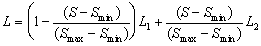# 4 Proposed Algorithm Scheme

## 4.1 Algorithm Scheme

• Create vector object to be filled by texture mapping fill style.
• project part of one of the basic 3D parametric surface (plane, cylinder, cone, sphere) on a vector objectĺs envelope to create basic tetragonal mesh
• assign texture coordinates to every point of mesh (semi-automatically)
• deform mesh using repulsive points or other warp algorithm.
• convert tetragonal mesh to triangular
• Map texture colors on each triangle.

The last step is critical for algorithm speed. For each point of triangle we can use algorithms described in chapter 2 based on barycentric coordinates or it is possible to extend scan line algorithm for polygon (triangle) filling ([FoDa91], [RuFe95]) to get barycentric coordinates faster. Barycentric coordinates of each pixel of scan line could be obtained by the linear interpolation of barycentric coordinates of first and last pixel of scan line. We can obtain barycentric coordinates of each scan line point by two additions of deltas, because we need only two barycentric coordinates. It's possible to show that the properties of map transforming barycentric coordinates to texture coordinates allow to use just two addition deltas again to get texture coordinates of each pixel of scan line.

## 4.2 Possible image quality enhancements

Only texture mapping can not generate shadow effects, but there are several possibilities to simulate shadows (analogous problem is opacity simulation)

Automatic:

• find the minimal and maximal mesh cellĺs area (Smin and Smax.).
• assign shadow levels to this areas (L1 and L2).
• use linear interpolation to compute shadow level of each mesh cell according the cell areas